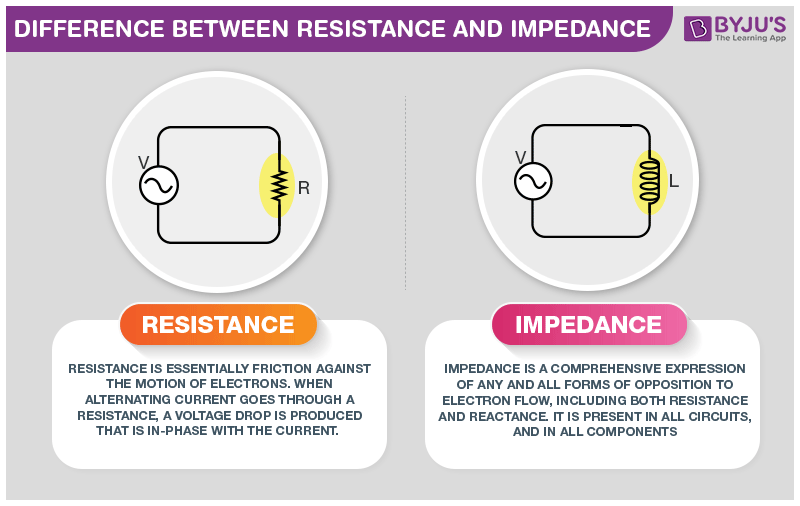# Difference Between Resistance and Impedance

Before knowing the difference between resistance and impedance, we should know that impedance is a concept related to AC whereas resistance is a concept related to DC. Their calculation of value is the same which is R=V/I.## Difference Between Resistance and Impedance

Resistance is caused due to the electrons in a conductor colliding with the ionic lattice of the conductor which results in the conversion of electrical energy into heat. It could be said that electrical resistance is the opposition to steady electric current. An ideal resistance does change with frequency when connected with DC.

Impedance is the measure of the nature of opposition of the AC electricity which is created due to inductance and capacitance. This opposition varies with the frequency. Very often, reactance and impedance are thought to be the same and are interchangeably used. It is important to understand that reactance is the resistance offered to the AC current by inductors and capacitors only while impedance is the sum of the resistance and reactance. In the table below, important differences between resistance and impedance are given.

Difference Between Resistance and Impedance

Resistance

Impedance

It is used in DC circuits.

It is used in AC circuits.

Resistance can be seen in both AC and DC circuits.

Impedance can be seen only in AC circuits.

It happens due to resistive elements.

It happens due to reactance and resistance.

It is represented by letter R.

It is represented by letter Z.

It is represented using real numbers such as 5.3 ohms.

It is represented using real and imaginary values such as R + ik.

It does not vary depending upon the frequency of DC current.

It varies according to the frequency of AC current.

It does not have a magnitude and phase angle.

It does have a phase angle and magnitude.

If kept in a electromagnetic field, it only shows the power dissipation.

If kept in a electromagnetic field, it shows power dissipation and energy stored.

These were some differences between Resistance and Impedance. If you wish to find out more, download BYJU’S The Learning App.

RELATED ARTICLES:

## Frequently Asked Questions – FAQs

### Is the resistance be seen only in AC circuits?

No, resistance can be seen in both AC and DC circuits.

### How is the impedance represented?

Impedance is represented using the alphabet ‘Z’.

### Does the value of resistance change when the frequency of DC current changes?

The value of resistance does not change upon the change in frequency of DC current.

### Do resistance have the magnitude and phase angle?

Resistance does not have a magnitude and phase angle.

### How does the impedance vary?

Impedence varies according to the frequency of AC current.
Test your knowledge on Resistance and impedance difference